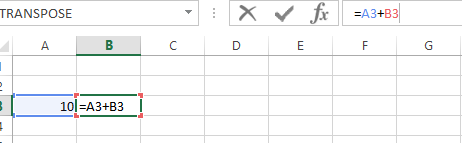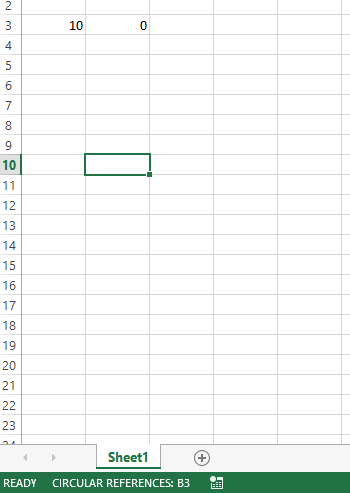### How To Get Rid Of Circular References In Excel

In this Article we will learn how to remove the circular references in an Excel file.

Let us understand what circular reference is. A circular reference happens and a formula directly of indirectly refers to its own cell. This causes the formula to use the result in the calculation and it runs in a loop and hence causes an error. When there are circular reference errors the Excel cannot do the other formula calculations automatically.

If you leave them in, Excel calculates each cell involved in the circular reference by using the results of the previous iteration. An iteration is a repeated recalculation until a specific numeric condition is met. By default, Excel stops calculating after 100 iterations or after all values in the circular reference change by less than 0.001 between iterations, unless you change the Excel option.

Lets create a circular reference now to look at it:

We wrote a value in cell A3 which is 10

In cell B3 I wrote a formula : = A3+B3 Now as we are already putting a formula in B3 and using the same in formula.On clicking enter/tab it gives the following error:On clicking ok it gives the wrong results = 0 and in the bottom it keeps showing warning:Now we will learn how to remove the circular references.

### How to enable / disable circular references in Excel

As noted earlier, iterative calculations are usually turned off in Excel be default (in this context, iteration is the repeated recalculation until a specific numeric condition is met). For circular formulas to work, you must enable iterative calculations in your Excel workbook.

In Excel 2019, Excel 2016, Excel 2013, and Excel 2010, click File > Options, go to Formulas, and select the Enable iterative calculation check box under the Calculation options section.

In Excel 2007, click Office button > Excel options > Formulas > Iteration area.

In Excel 2003 and earlier, the Iterative Calculation option resides under Menu > Tools > Options > Calculation tab. When you turn on iterative calculations, you must specify the following two options:

1. Maximum Iterations box – specifies how many times the formula should recalculate. The higher the number of iterations, the more time the calculation takes.
2. Maximum Change box – specifies the maximum change between calculation results. The smaller the number, the more accurate result you get and the more time Excel takes to calculate the worksheet. The default settings are 100 for Maximum Iterations, and 0.001 for Maximum Change. What it means is that Microsoft Excel will stop calculating your circular formula after 100 iterations or after a less than 0.001 change between iterations, whichever comes first.

## Template

```Further reading: